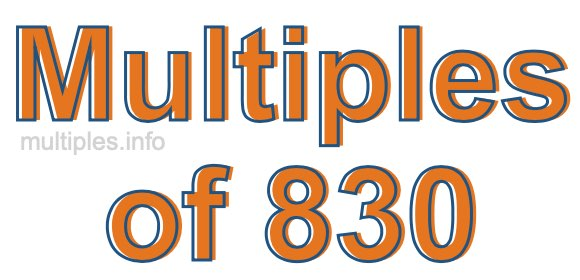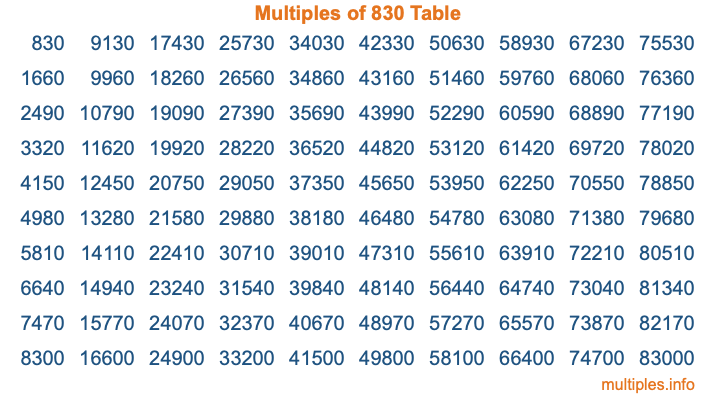Multiples of 830Welcome to the Multiples of 830 page. Here we will first teach you everything you will ever need to know about the multiples of 830, and then give you a study guide summary of everything we taught you to make sure you remember it all. Use this page to look up facts and learn information about the multiples of 830. This page will make you a multiples of eight hundred thirty expert!

Definition of Multiples of 830
Multiples of 830 are all the numbers that when divided by 830 equal an integer. Each of the multiples of 830 are called a multiple. A multiple of 830 is created by multiplying 830 by an integer.

Therefore, to create a list of multiples of 830, you start with 1 multiplied by 830, then 2 multiplied by 830, then 3 multiplied by 830, and so on for as long as you want. Thus, the list of the first five multiples of 830 is 830, 1660, 2490, 3320, and 4150. To see a larger list of multiples of 830, see the printable image of Multiples of 830 further down on this page. We also have a category where you can choose any nth multiple of 830.

Multiples of 830 Checker
The Multiples of 830 Checker below checks to see if any number of your choice is a multiple of 830. In other words, it checks to see if there is any number (integer) that when multiplied by 830 will equal your number. To do that, we divide your number by 830. If the the quotient is an integer, then your number is a multiple of 830.

Is  a multiple of 830?

Least Common Multiple of 830 and ...
A Least Common Multiple (LCM) is the lowest multiple that two or more numbers have in common. This is also called the smallest common multiple or lowest common multiple and is useful to know when you are adding our subtracting fractions. Enter one or more numbers below (830 is already entered) to find the LCM.

Check out our LCM Calculator if you need more details about the Least Common Multiple or if you need the LCM for different numbers for adding and subtraction fractions.

nth Multiple of 830
As we stated above, 830 is the first multiple of 830, 1660 is the second multiple of 830, 2490 is the third multiple of 830, and so on. Enter a number below to find the nth multiple of 830.

th multiple of 830

Multiples of 830 vs Factors of 830
830 is a multiple of 830 and a factor of 830, but that is where the similarities end. All postive multiples of 830 are 830 or greater than 830. All positive factors of 830 are 830 or less than 830.

Below is the beginning list of multiples of 830 and the factors of 830 so you can compare:

Multiples of 830: 830, 1660, 2490, 3320, 4150, etc.

Factors of 830: 1, 2, 5, 10, 83, 166, 415, 830

As you can see, the multiples of 830 are all the numbers that you can divide by 830 to get a whole number. The factors of 830, on the other hand, are all the whole numbers that you can multiply by another whole number to get 830.

It's also interesting to note that if a number (x) is a factor of 830, then 830 will also be a multiple of that number (x).

Multiples of 830 vs Divisors of 830
The divisors of 830 are all the integers that 830 can be divided by evenly. Below is a list of the divisors of 830.

Divisors of 830: 1, 2, 5, 10, 83, 166, 415, 830

The interesting thing to note here is that if you take any multiple of 830 and divide it by a divisor of 830, you will see that the quotient is an integer.

Multiples of 830 Table
Below is an image of the first 100 multiples of 830 in a table. The table is in chronological order, column by column. The first column has the first ten multiples of 830, the second column has the next ten multiples of 830, and so on.The Multiples of 830 Table is also referred to as the 830 Times Table or Times Table of 830. You are welcome to print out our table for your studies.

Negative Multiples of 830
Although not often discussed or needed in math, it is worth mentioning that you can make a list of negative multiples of 830 by multiplying 830 by -1, then by -2, then by -3, and so on, to get the following list of negative multiples of 830:

-830, -1660, -2490, -3320, -4150, etc.

Multiples of 830 Summary
Below is a summary of important Multiples of 830 facts that we have discussed on this page. To retain the knowledge on this page, we recommend that you read through the summary and explain to yourself or a study partner why they hold true.

There are an infinite number of multiples of 830.

A multiple of 830 divided by 830 will equal a whole number.

830 divided by a factor of 830 equals a divisor of 830.

The nth multiple of 830 is n times 830.

The largest factor of 830 is equal to the first positive multiple of 830.

830 is a multiple of every factor of 830.

830 is a multiple of 830.

A multiple of 830 divided by a divisor of 830 equals an integer.

830 divided by a divisor of 830 equals a factor of 830.

Any integer times 830 will equal a multiple of 830.

Multiples of a Number
Here you can get the multiples of another number, all with the same attention to detail as we did for multiples of 830 on this page.

Multiples of
Multiples of 831
Did you find our page about multiples of eight hundred thirty educational? Do you want more knowledge? Check out the multiples of the next number on our list!

Copyright  |   Privacy Policy  |   Disclaimer  |   Contact